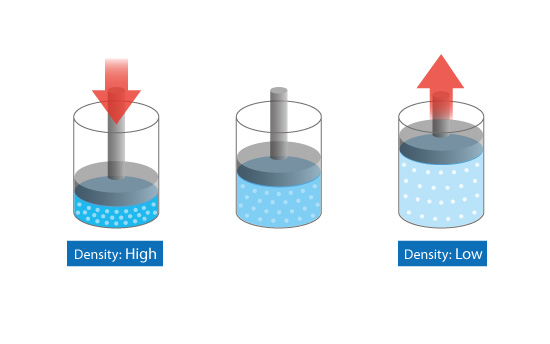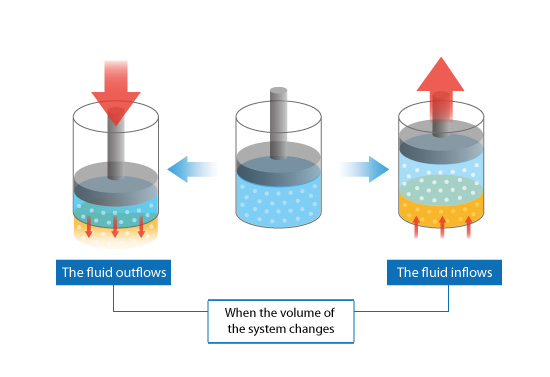# Basic Course of Thermo-Fluid Analysis 06: Chapter 3 Basics of Flow - 3.2.1 Compressible/incompressible fluids

#### 3.2 Characteristics of flows

This chapter introduces some of the important characteristics of a flow. The difference in flow characteristics may affect how the flow is analyzed. In addition, knowing the flow characteristics is very important when evaluating the validity of an obtained result.

#### 3.2.1 Compressible/incompressible fluids

Compression and expansion are important characteristics of a fluid. Remember that a fluid can be a liquid or a gas. If compression and expansion have a significant effect on the fluid density (kg/m3), the fluid is called a compressible fluid. Consider a simple example of a gas in a cylinder as shown in Figure 3.10. The cylinder is sealed so that gas cannot enter or escape. The fluid volume changes as the piston moves. However, the mass of the system does not change because the gas is not allowed to enter or leave the cylinder. Therefore, the fluid density must change because of the change in volume.Figure 3.10: Compressible fluid

On the other hand, when compression and expansion do not significantly affect the fluid density, the fluid is called an incompressible fluid. The volume of an incompressible fluid does not change and its density is treated as a constant. Consider a liquid in a cylinder. If the cylinder is sealed, the piston will stop moving once it contacts the liquid. As the piston retracts, an empty space is created above the liquid surface. The amount of space (volume) the liquid occupies does not change (actually the volume does change but the change is very tiny). Since the amount of the liquid is almost unchanged, the fluid density (kg/m3) is constant. Liquids are always considered to be incompressible fluids, as density changes caused by pressure and temperature are small.

While intuitively gases may always seem to be incompressible fluids if the gas is permitted to move, a gas can be treated as being incompressible if its change in density is small. Consider the cylinder filled with a gas as shown in Figure 3.11. Ports are added to the cylinder that permits gas to enter or leave the cylinder. As the piston pushes down, the gas flows out of the port because the volume of the cylinder decreases. The amount of mass of the gas also decreases proportionally, and the density of the gas (kg/m3) in the cylinder is unchanged. When the piston retracts, the volume of the system increases, gas (mass) enters through the port and the density of the gas (kg/m3) remains again essentially constant. In this situation, the gas behaves as an incompressible fluid. In a strict sense, a completely incompressible fluid does not exist. However, when the density changes due to pressure (the movement of the piston applies pressure to the fluid in the cylinder) or temperature is small, approximating a fluid as an incompressible fluid can greatly simplify calculations.Figure 3.11: Incompressible fluid

One measure of the degree of compressibility of a gas is the Mach number M of the flow. The Mach number is the ratio of the fluid velocity to the speed of sound. When M < approx. 0.3, a fluid can be treated as incompressible. For an air temperature of 20°C, the speed of sound is approximately 340 m/s. Therefore, if the fluid velocity is 100 m/s or greater, compressibility should be considered in the calculations. For fluid velocities less than 100 m/s, the fluid can be considered incompressible. In addition, if the fluid temperature changes significantly (this is different than the fluid being at a constant high or low temperature), the fluid density will also change substantially during volume expansion or compression. In this case, the fluid may also be treated as a compressible fluid.